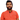# Learn Pain LessHomeOur TeamContact
Java
If statement in java, how to usePawneshwer Gupta
February 16, 2015
1 minIf statement is used when we want to check a condition. We check a condition to prevent our program from sudden crash. Mostly our app crash due to lack of validation. If we validate value before using, then we will not face any error or exception.

For example, if we want to device two values, but 2nd value is 0 then our program will crash saying mathematical exception, because in java we can’t divide any number with 0.

To prevent our program from crash we can apply validation on our code. We will check if divisible value is 0 them don’t divide. We can do this using below code.

Suppose we have two variables

`.css-1baulvz{display:inline-block;}int a, b;//Anda = 15;b = 0;`

and if we divide a with b then we will get error that we can’t divide any number with 0.

So apply this condition

`if ( b > 0) {   // Now divide variables.   int sum = a/b; } else {   // Print message that b is 0.   System.out.println("b is 0"); }`

So in this condition we checked that if b is greater than 0 then divide variables. Otherwise display message that b is 0.

Now our app will not crash because when value of b will be 0 then division will be skipped.

If condition will return true then if part will be executed, otherwise else part will be executed.

Condition inside if parentheses will be Boolean. Which means that condition will return true or false. We can’t pass string, int or any other value inside if parentheses.

For example, we can’t use

```if (10){  //10 is int value but if() require Boolean value. We can't use this  // but below code is correct.}
if(a == 10){   //This condition is correct.}```

We'll send you the best of our blog just once a month. We promise.

startif

## Software Developer

Pawneshwer Gupta works as a software engineer who is enthusiastic in creating efficient and innovative software solutions.

Python
Flutter
Laravel
NodeJS

## Related PostsWorking with Files, Folders java
March 25, 2015
1 min